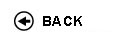Politecnico di Torino
16ACFLZ, 16ACFLN, 16ACFLS, 16ACFLX, 16ACFMA, 16ACFMB, 16ACFMC, 16ACFMH, 16ACFMK, 16ACFMN, 16ACFMO, 16ACFMQ, 16ACFNL, 16ACFNM, 16ACFNX, 16ACFNZ, 16ACFOA, 16ACFOD, 16ACFPC, 16ACFPI, 16ACFPL, 16ACFQR
Mathematical analysis I
1st degree and Bachelor-level of the Bologna process in Aerospace Engineering - Torino
1st degree and Bachelor-level of the Bologna process in Automotive Engineering - Torino
1st degree and Bachelor-level of the Bologna process in Material Engineering - Torino
Espandi...
 Teacher Status SSD Les Ex Lab Tut Years teaching Adami Riccardo O2 MAT/05 60 40 0 0 8 Camporesi RobertoAC MAT/05 60 40 0 0 11 Ceragioli Francesca MariaA2 MAT/05 60 40 0 0 11 Chiado' Piat ValeriaO2 MAT/05 60 40 0 0 19 Codegone Marco60 40 0 60 14 Fagnani FabioPO MAT/05 60 40 0 0 11 Mazzi LuisaAC MAT/05 60 40 0 0 22 Nicola Fabio O2 MAT/05 60 40 0 0 10 Pandolfi Luciano60 40 0 0 12 Pandolfi Luciano60 40 0 0 12 Pellerey FrancoPO MAT/06 60 40 0 0 3 Serra EnricoPO MAT/05 60 40 0 0 8 Tabacco Anita MariaPO MAT/05 60 40 0 0 23 Tilli PaoloPO MAT/05 60 40 0 0 9 Cortese Paolo60 40 0 0 12 Cortese Paolo60 40 0 0 12 Rolando Sergio60 40 0 0 3 Dambrosio Walter60 40 0 0 8
 SSD CFU Activities Area context MAT/05 10 A - Di base Formazione matematica di base
Esclusioni:
04KWQ
 Subject fundamentals This course represents a bridge between high school and college ways of teaching and learning. The main goal of the course is to provide the students with tools to understand and elaborate logical arguments, from simple to more elaborate ones, and to introduce the elements of differential and integral calculus for functions of one variable with applications to ordinary differential equations of the first order and of the second order linear case. Complex numbers will also be introduced and applied to the solutions of linear equations of the second order with constant coefficients. Expected learning outcomes Ability to follow a chain of logical arguments. Essentials of differential and integral calculus for functions of one variable. Computational ability. Prerequisites / Assumed knowledge Numbers, equations and inequalities, analytical geometry and trigonometry. First properties of elementary functions. Contents Preliminaries: sets and their operations. Number sets, maxima, minima and extrema. Completeness properties of real numbers and consequences. Functions: surjectivity and injectivity; composition of functions, inverse functions. Functions of a real variable: elementary functions, monotonic functions and inverse functions. (About 15 hours) Limits and continuity: theory of limits of functions and sequences. Continuous functions and related theorems. Order and comparison theorems. Algebra of limits. Indeterminate forms. Local comparison of functions. Landau symbols. Infinite and infinitesimal functions. Order of an infinity and of an infinitesimal, principal part (with respect to a test function). Asymptotes. The number e. Trigonometric and exponential fundamental limits. Functions which are continuous on an interval: existence of zeros and of maxima and minima. (About 24 hours) Derivatives: geometrical and physical meaning. Computation of derivatives. List of fundamental derivatives. Derivatives and continuity. Lack of derivatives, extremal and critical points. Fermat Theorem. Functions with derivatives on an interval and fundamental theorems of differential calculus (Rolle and Lagrange theorems) and consequences. de L'Hospital rule. Taylor formula and fundamental MacLaurin formulas. Use of Taylor expansions in the local analysis of functions: comparison, extrema and convexity. (About 23 hours) Primitives: rules of computation; primitives of rational functions. Indefinite integral. Riemann integral and its properties: monotonicity, additivity, linearity. Integral mean value. Classes of integrable functions. Fundamental theorem of integral calculus: relations between definite integrals and primitives. Improper integrals: definitions and convergence tests. (About 21 hours) Complex numbers and differential equations: algebraic and trigonometric forms of complex numbers. Real and imaginary parts, modulus and argument. Roots of complex numbers; Fundamental theorem of Algebra. Exponentials of complex numbers and Euler formulas. Ordinary differential equations: Cauchy problem. First order ordinary differential equations, with separable variables or linear. Second order differential equations, linear with constant coefficients. (About 17 hours) Delivery modes Both lessons and exercise classes will be given. Assessment and grading criteria The exam consists of a test and of a written and an oral part. The test (on computer, one hour long) consists of 20 multiple-choice questions of both theoretical and practical type. The students who have passed the test, can decide either to conclude the exam with only that part or they can take also a written part containing exercises and theory. In case this is passed, an oral exam will follow, upon the request of the student or of the teacher. Programma definitivo per l'A.A.2012/13